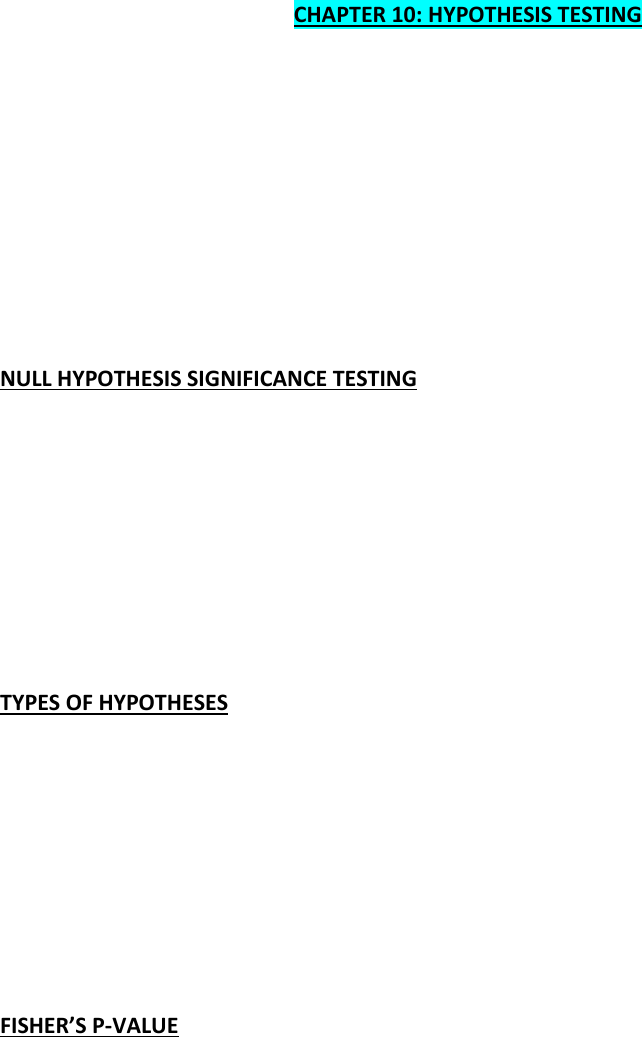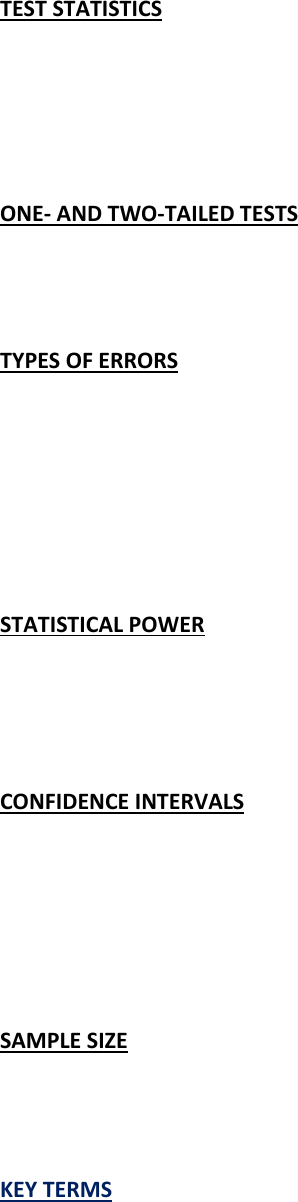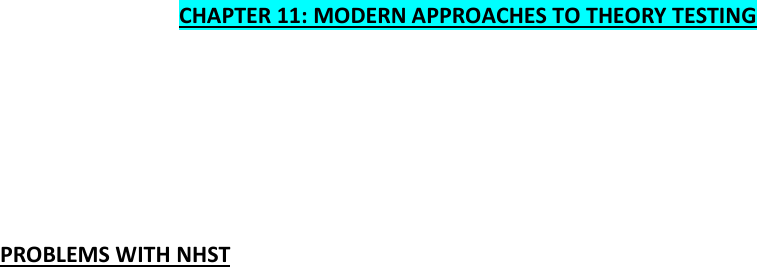Textbook Notes (280,000)
CA (170,000)
McMaster (10,000)
PNB (70)
Chapter 10-12

# PNB 3XE3 Chapter Notes - Chapter 10-12: Null Hypothesis, Statistical Power, Confidence Interval

Department
Psychology, Neuroscience & Behaviour
Course Code
PNB 3XE3
Professor
Rutherford
Chapter
10-12

This preview shows pages 1-3. to view the full 11 pages of the document.CHAPTER 10: HYPOTHESIS TESTING
THINGS TO KNOW
Null hypothesis significance testing
Types of hypothesis
Fisher’s p-value
Test statistics
One- and two-tailed tests
Type I and Type II errors
Statistical power
Confidence intervals
Sample size and statistical significance
NULL HYPOTHESIS SIGNIFICANCE TESTING
Evaluating evidence using competing hypotheses
Using probabilities to evaluate evidence
a. What is the probability of getting this data set if the Null hypothesis were true?
Method
1. Make predictions
2. Collect data
3. Assume the null hypothesis
4. Calculate the probability (p-value) of getting the data if the null hypothesis were true
5. Draw conclusions
TYPES OF HYPOTHESES
1. Alternative hypothesis
1. Experimental hypothesis
2. H1
3. The prediction from your theory
4. There is an effect
2. Null hypothesis
1. There is no effect
a. The data gathered are a result of a chance distribution
2. H0
FISHER’S P-VALUE
1. The level of probability at which you are prepared to believe the experimental
hypothesis
2. The level of probability at which you are prepared to reject the null hypothesis
3. The data you have observed are unlikely to have occurred by chance
4. P-value
5. a
6. often .05, sometimes .01

Only pages 1-3 are available for preview. Some parts have been intentionally blurred.TEST STATISTICS
1. How good is the hypothesis compared to how bad the error is
2. Signal/noise
3. Effect/error
4. Parameter estimate (b) / standard error of b
5. Examples: t, F, X2
ONE- AND TWO-TAILED TESTS
1. Do you have a prediction?
2. Must be decided before data collection
3. You would miss any “effect” in the non-predicted direction
TYPES OF ERRORS
1. Type I
1. Believe there is an effect when there is not
2. Probability is equal to the a-level
3. Be mindful of the family-wise error rate!
2. Type II
1. Believe in the null hypothesis when there is an effect
2. Probability is equal to the B-level
STATISTICAL POWER
1. The ability of a test statistic to detect an effect
1-B
2. Sensitive to sample size
3. All else equal, power is the same across 1-tailed tests and 2-tailed tests
CONFIDENCE INTERVALS
A measure of variability around the mean
A 95% confidence interval = the mean plus/minus 1.96* the standard error of the
mean
Moderate overlap is half the length of the average Margin of error (MOE)
P=0.05 means an overlap of a quarter of the confidence interval (provided the
confidence intervals are the same length)
SAMPLE SIZE
A larger sample will decrease the standard error of the mean
o Hence smaller confidence interval
It will not decrease the standard deviation
KEY TERMS
a level: probability of making a Type I error (value is usually 0.05)
B level: probability of making a Type II error (Cohen suggests a maximum value of 0.02)

Only pages 1-3 are available for preview. Some parts have been intentionally blurred.Experimental-wise error rate: probability of making a Type I error in an experiment
involving one of more statistical comparisons when the null hypothesis is true in each
case
Family-wise error rate: probability of making a Type I error in any family of tests when
the null hypothesis is true in each case. The ‘family of tests’ can be loosely defined as a
set of tests conducted on the same data set and addressing the same empirical
question.
Variables: measured constructs that vary across entities in the sample
Parameters: estimated from the data and are usually constructs believed to represent
some fundamental truth about the relations between variables in the model
CHAPTER 11: MODERN APPROACHES TO THEORY TESTING
THINGS TO KNOW
Problems with NHST
Effect sizes
Meta-analysis
Bayesian approaches
PROBLEMS WITH NHST
Significance is not the same as importance
o An unimpressive result can be significant if the sample is large enough
o Or large effects can be missed if the sample is too small
Even a rejection of the null hypothesis is inherently probabilistic
o Yet our conclusions are all-or-none
o Even the logic doesn’t hold
Because significant testing is based on probabilities you can’t use these sorts of logical
statements to rule out the null hypothesis
“if someone plays guitar then it is highly unlikely that he or she plays in
The Reality Enigma.
The person plays in The Reality Enigma.
Therefore, this person is highly unlikely to play guitar”
o This would be unproblematic:
if the null hypothesis is correct, then this test statistic cannot occur.
This test statistic has occurred. Therefore, the null hypothesis is
false”
o BUT this is what you have
“if the null hypothesis is correct, then this test statistic is highly unlikely
This test statistic has occurred. Therefore, the null hypothesis is
highly unlikely”
Anyway, even if the null hypothesis is false, how do you know that YOUR experimental
hypothesis is true?
o SOLUTIONS TO THESE PROBLEMS